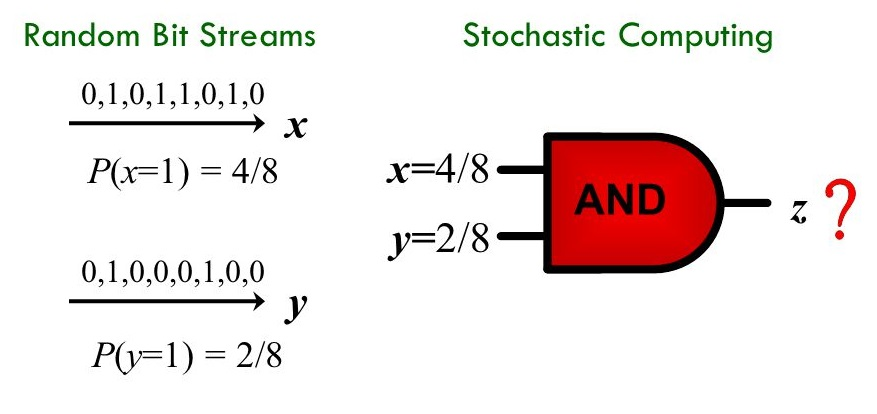# Deep Convolutional Neural Networks (DCNNs) For Embedded and Portable Systems

Stochastic Computing (SC), which uses a bit-stream to represent a number within [-1, 1] by counting the number of ones in the bit-stream, has high potential for implementing CNNs with ultra-low hardware footprint. Since multiplications and additions can be calculated using AND gates and multiplexers in SC, significant reductions in power (energy) and hardware footprint can be achieved compared to the conventional binary arithmetic implementations.

In this project we will implement the basic elements of CNN using Stochastic computing concepts. The implementation of the elements will be in Systemverilog or VHDL, followed by application to a network example for validation and power/performance analysis.Project description:

With the recent advance of wearable devices and Internet of Things (IoTs), it becomes attractive to implement the Deep Convolutional Neural Networks (DCNNs) in embedded and portable systems. Currently, executing the software-based DCNNs requires high-performance and high-power servers.

Stochastic Computing (SC), which uses a bit-stream to represent a number within [-1, 1] by counting the number of ones in the bit-stream, has high potential for implementing CNNs with ultra-low hardware footprint. Since multiplications and additions can be calculated using AND gates and multiplexers in SC, significant reductions in power (energy) and hardware footprint can be achieved compared to the conventional binary arithmetic implementations.

In this project we will implement the basic elements of CNN using Stochastic computing concepts. The implementation of the elements will be in Systemverilog or VHDL, followed by application to a network example for validation and power/performance analysis.

The work will be performed using Cadence design tools based on latest process technology models. The students will gain exposure to CNN implementation field, knowledge of advanced computing techniques, as well as low-power logical design.

Recommended: Logic Design

Example reference:

https://arxiv.org/pdf/1611.05939.pdf